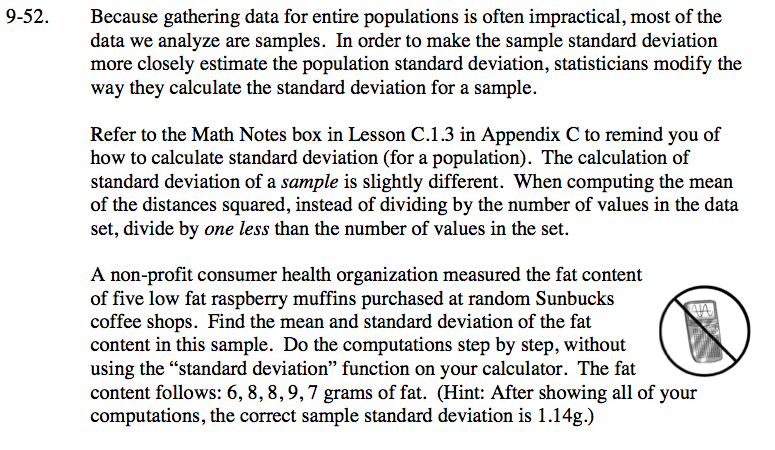Home > CCA2 > Chapter 9 > Lesson 9.2.1 > Problem9-52

9-52.
1. Because gathering data for entire populations is often impractical, most of the data we analyze are samples. In order to make the sample standard deviation more closely estimate the population standard deviation, statisticians modify the way they calculate the standard deviation for a sample.

2. Refer to the Math Notes box in Lesson C.1.3 in Appendix C to remind you of how to calculate standard deviation (for a population). The calculation of standard deviation of a sample is slightly different. When computing the mean of the distances squared, instead of dividing by the number of values in the data set, divide by one less than the number of values in the set.

3.A non-profit consumer health organization measured the fat content of five low fat raspberry muffins purchased at random Sunbucks coffee shops. Find the mean and standard deviation of the fat content in this sample. Do the computations step by step, without using the “standard deviation” function on your calculator. The fat content follows: 6, 8, 8, 9, 7 grams of fat. (Hint: After showing all of your computations, the correct sample standard deviation is 1.14g.) Homework Help ✎Find the mean.

mean = 7.6

Find the distances to the mean from each data point.

6 − 7.6 = −1.6
8 − 7.6 = ?
8 − 7.6 = ?
9 − 7.6 = ?
7 − 7.6 = ?

Square the distances.

−1.6 distance squared = 2.56
.4 distance squared = .16
?
?
?

Find the mean of the distances squared.
Since this is a sample, divide by one less than the number of data items.